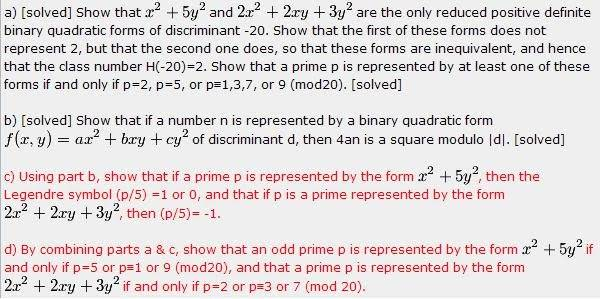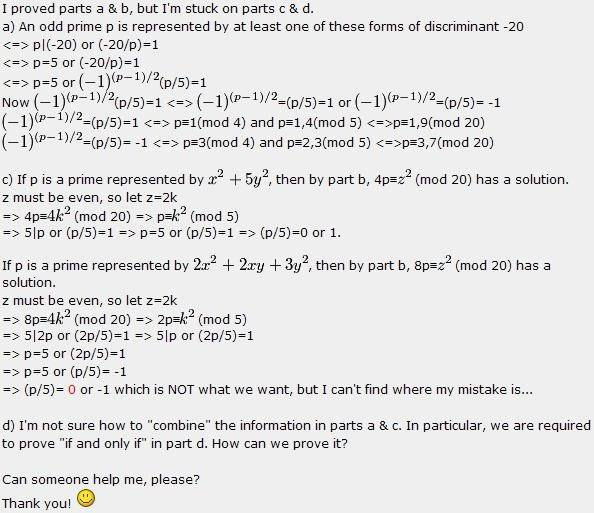# Number theory: Binary Quadratic Forms

kingwinnerP.S. I'm not sure where to post this question, in particular I can't find a number theory forum on the coursework section for textbook problems. Please move this thread to the appropriate forum if this is not where it should belong to. Thanks!

Last edited:

kingwinnerrobert Ihnot
All I can tell you is that if x^2 + 5y^2 ==0 Mod p. Then it follows that (x/y)^2 ==-5 Mod p. But looking at that Mod 4, we have the form x^2 + y^2 ==1 Mod 4, because they can not be both even or odd, otherwise p would be divisible by 2.
The form 4k+1 has -1 as a quadratic residue. So we have a solution to u^2 ==5 Mod p. From the form (p/5)=1, we have the solution v^2 ==+/-1 Mod 5. To fulfill both these cases, we have 20k+1, 20k+9.

By looking at the various cases we can see that in the form 2x^2+2xy +3Y^2 =p, we know that y must be odd, and that for either x is odd or even we arrive at p== 3y^2==3 Mod 4. Thus -1 is not a quadratic residue Mod p. As you have written (p/5) = -1. So p is not a quadratic residue Mod 5. Thus p is of the form p= 5k+/- 2 Mod 5. We have the result p = 20k+ 3 or 7.

But you may already know this.
.

Last edited:
Gold Member
P.S. I'm not sure where to post this question, in particular I can't find a number theory forum on the coursework section for textbook problems. Please move this thread to the appropriate forum if this is not where it should belong to. Thanks!

Questions about number theory may be posted in the Calculus & Beyond section in the coursework forum.

Petek

kingwinner
So for part c, I think we have to show that the case p=5 is impossible.

In short, my question just translates to the following:
How can we prove that $$2x^{2}+2xy+3y^{2} =5$$ has no integer solutions?

Thanks!

robert Ihnot
You might get that by brute force. We know that both x and y can not be positive since even x=y=1 will sum to 7. It doesn't matter which is negative, but we rewrite the equation as 2x^2-2xy+3y^2. Y must be odd and if as large as 3, we have 2x^2-6x=-22. This gives x^2-3x = 11. Which gives x(x-3) =11, which is a prime and so this is impossible.

Possibly, by proceeding in this way, we can figure this thing out.

Once we know a few facts from the above, we can look at the matter as a congruence modulo 4. In this case y and all odd squares are congruent to 1. So the matter reduces to 2X^2 +2xy==-2 Mod 4, and then to x^2+xy ==1 Mod 2. So x must be odd, x^2==1 Mod 2, and thus xy==0 Mod 2, which is impossible. Maybe that will work!

Last edited:
kingwinner
Claim: $$2x^{2}+2xy+3y^{2}=5$$ has no integer solutions

If we complete the square, would that help?
$$(x+y)^{2}+x^{2}+2y^{2} = 5$$

Is it possible to eliminate some cases from here and show the remaining cases do not give 5? If so, how?

Martin Rattigan
Claim: $$2x^{2}+2xy+3y^{2}=5$$ has no integer solutions

If we complete the square, would that help?
$$(x+y)^{2}+x^{2}+2y^{2} = 5$$

Is it possible to eliminate some cases from here and show the remaining cases do not give 5? If so, how?

(Post by originator, so I assume it was a question rather than a hint.)

The answer then drops out. You can't have $x=y=0(5)$ otherwise $5^2\mid$ LHS but $5^2\nmid$ RHS.

So $x\neq 0(5)$, $y\neq 0(5)$ and $x+y\neq 0(5)$.

So $(x+y)^{2}+x^{2}+2y^{2}\geq 7$.